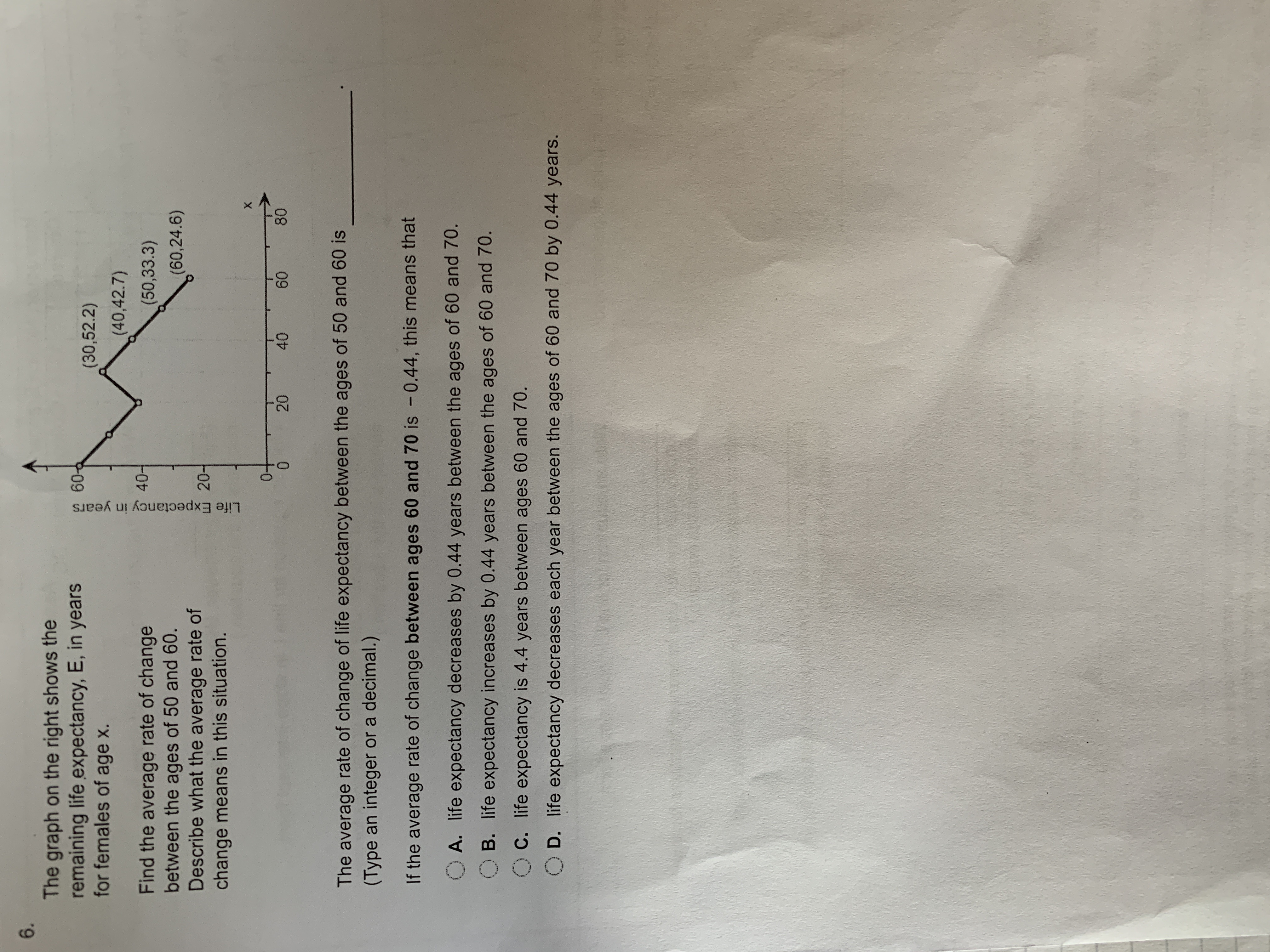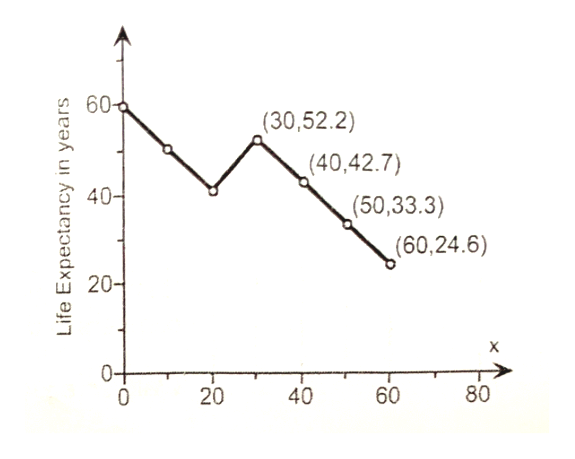# 6.The graph on the right shows theremaining life expectancy, E, in yearsfor females of age60-d(30,52.2)(40,42.7)40Find the average rate of changebetween the ages of 50 and 60.Describe what the average rate ofchange means in this situation.(50,33.3)(60,24.6)20Xet ato8020400The average rate of change of life expectancy between the ages of 50 and 60 is(Type an integer or a decimal.)If the average rate of change between ages 60 and 70 is - 0.44, this means thatA. life expectancy decreases by 0.44 years between the ages of 60 and 70.B. life expectancy increases by 0.44 years between the ages of 60 and 70.OC. life expectancy is 4.4 years between ages 60 and 70.O D. life expectancy decreases each year between the ages of 60 and 70 by 0.44 years.Life Expectancy in years60

Question

Can someone help me with this problem ?help_outlineImage Transcriptionclose6. The graph on the right shows the remaining life expectancy, E, in years for females of age 60-d (30,52.2) (40,42.7) 40 Find the average rate of change between the ages of 50 and 60. Describe what the average rate of change means in this situation. (50,33.3) (60,24.6) 20 X et ato 80 20 40 0 The average rate of change of life expectancy between the ages of 50 and 60 is (Type an integer or a decimal.) If the average rate of change between ages 60 and 70 is - 0.44, this means that A. life expectancy decreases by 0.44 years between the ages of 60 and 70. B. life expectancy increases by 0.44 years between the ages of 60 and 70. OC. life expectancy is 4.4 years between ages 60 and 70. O D. life expectancy decreases each year between the ages of 60 and 70 by 0.44 years. Life Expectancy in years 60 fullscreen
check_circleExpert Solution
Step 1

Let in the given graph life expectancy represents y-axis and time represents the x-axis.help_outlineImage Transcriptionclose60 (30,52.2) (40,42.7) 40 (50,33.3) (60,24.6) 20- X 80 60 40 Life Expectancy in years |20 fullscreen
Step 2

The formula of average rate of change is given by:

Step 3

The average rate of change between the ages of 50 and 60 is given by taking x1 = 50, x2 = 60 and their corresponding y1 = 33.3, ...

### Want to see the full answer?

See Solution

#### Want to see this answer and more?

Solutions are written by subject experts who are available 24/7. Questions are typically answered within 1 hour*

See Solution
*Response times may vary by subject and question
Tagged in

### Applications of Mathematics